## # 周一

1. 确定dp数组含义

dp[i]：考虑下标i（包括i）以内的房屋，最多可以偷窃的金额为dp[i]

1. 确定递推公式

dp[i] = max(dp[i - 2] + nums[i], dp[i - 1]);

1. dp数组如何初始化
``````vector<int> dp(nums.size());
dp = nums;
dp = max(nums, nums);
``````
1
2
3
1. 确定遍历顺序

1. 举例推导dp数组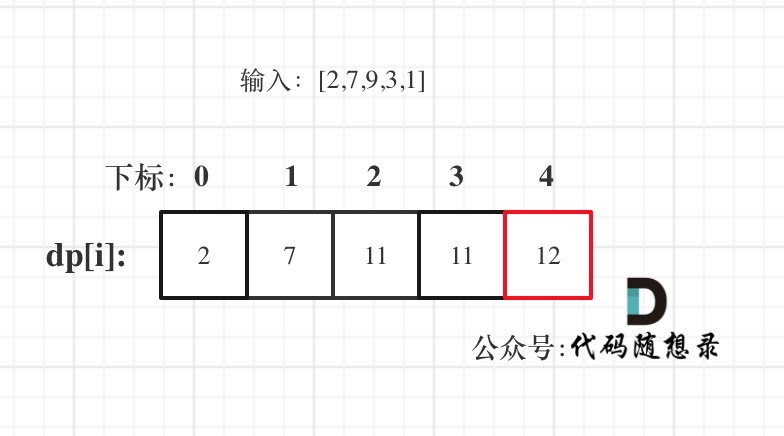## # 周二

• 情况一：考虑不包含首尾元素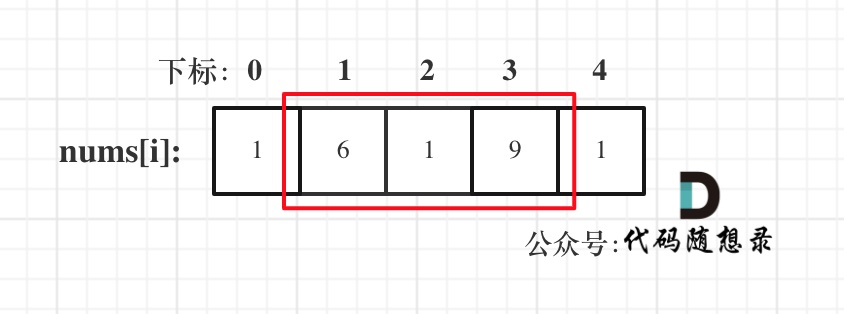• 情况二：考虑包含首元素，不包含尾元素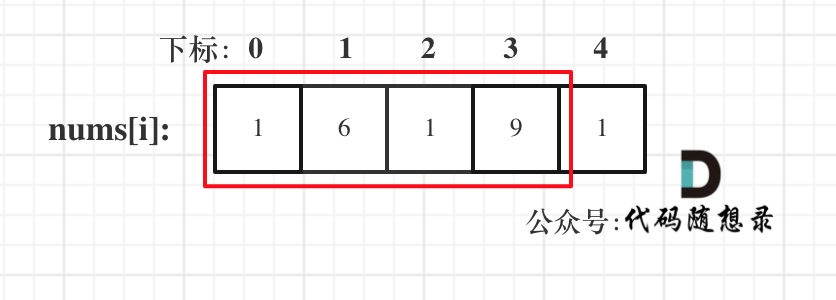• 情况三：考虑包含尾元素，不包含首元素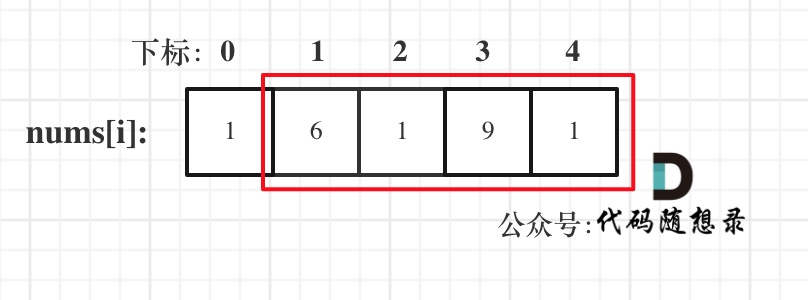## # 周三

``````class Solution {
public:
int rob(TreeNode* root) {
if (root == NULL) return 0;
if (root->left == NULL && root->right == NULL) return root->val;
// 偷父节点
int val1 = root->val;
if (root->left) val1 += rob(root->left->left) + rob(root->left->right); // 跳过root->left，相当于不考虑左孩子了
if (root->right) val1 += rob(root->right->left) + rob(root->right->right); // 跳过root->right，相当于不考虑右孩子了
// 不偷父节点
int val2 = rob(root->left) + rob(root->right); // 考虑root的左右孩子
return max(val1, val2);
}
};
``````
1
2
3
4
5
6
7
8
9
10
11
12
13
14

``````class Solution {
public:
unordered_map<TreeNode* , int> umap; // 记录计算过的结果
int rob(TreeNode* root) {
if (root == NULL) return 0;
if (root->left == NULL && root->right == NULL) return root->val;
if (umap[root]) return umap[root]; // 如果umap里已经有记录则直接返回
// 偷父节点
int val1 = root->val;
if (root->left) val1 += rob(root->left->left) + rob(root->left->right); // 跳过root->left
if (root->right) val1 += rob(root->right->left) + rob(root->right->right); // 跳过root->right
// 不偷父节点
int val2 = rob(root->left) + rob(root->right); // 考虑root的左右孩子
umap[root] = max(val1, val2); // umap记录一下结果
return max(val1, val2);
}
};
``````
1
2
3
4
5
6
7
8
9
10
11
12
13
14
15
16
17

1. 确定递归函数的参数和返回值
``````vector<int> robTree(TreeNode* cur) {
``````
1

dp数组含义：下标为0记录不偷该节点所得到的的最大金钱，下标为1记录偷该节点所得到的的最大金钱。

1. 确定终止条件

``````if (cur == NULL) return vector<int>{0, 0};
``````
1
1. 确定遍历顺序

``````// 下标0：不偷，下标1：偷
vector<int> left = robTree(cur->left); // 左
vector<int> right = robTree(cur->right); // 右
// 中

``````
1
2
3
4
5
1. 确定单层递归的逻辑

``````vector<int> left = robTree(cur->left); // 左
vector<int> right = robTree(cur->right); // 右

// 偷cur
int val1 = cur->val + left + right;
// 不偷cur
int val2 = max(left, left) + max(right, right);
return {val2, val1};
``````
1
2
3
4
5
6
7
8
1. 举例推导dp数组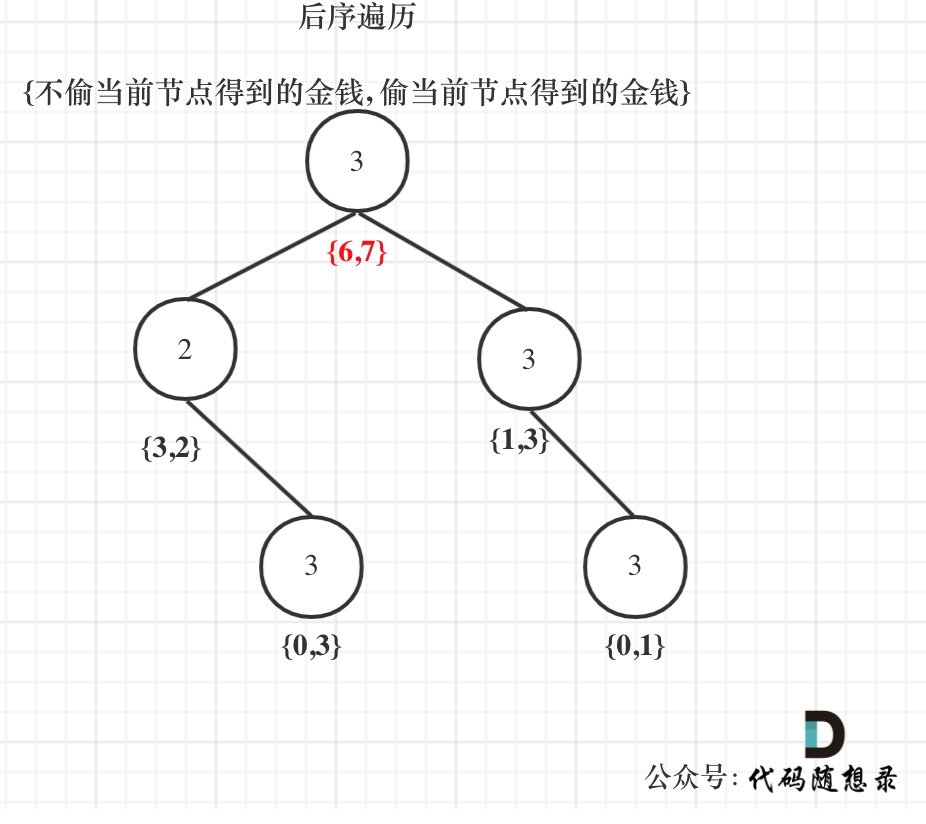“树形贪心”词汇从此诞生，来自「代码随想录」

## # 周四

``````class Solution {
public:
int maxProfit(vector<int>& prices) {
int result = 0;
for (int i = 0; i < prices.size(); i++) {
for (int j = i + 1; j < prices.size(); j++){
result = max(result, prices[j] - prices[i]);
}
}
return result;
}
};
``````
1
2
3
4
5
6
7
8
9
10
11
12
• 时间复杂度：O(n^2)
• 空间复杂度：O(1)

``````class Solution {
public:
int maxProfit(vector<int>& prices) {
int low = INT_MAX;
int result = 0;
for (int i = 0; i < prices.size(); i++) {
low = min(low, prices[i]);  // 取最左最小价格
result = max(result, prices[i] - low); // 直接取最大区间利润
}
return result;
}
};
``````
1
2
3
4
5
6
7
8
9
10
11
12
• 时间复杂度：O(n)
• 空间复杂度：O(1)

``````// 版本一
class Solution {
public:
int maxProfit(vector<int>& prices) {
int len = prices.size();
vector<vector<int>> dp(len, vector<int>(2));
dp -= prices;
dp = 0;
for (int i = 1; i < len; i++) {
dp[i] = max(dp[i - 1], -prices[i]);
dp[i] = max(dp[i - 1], prices[i] + dp[i - 1]);
}
return dp[len - 1];
}
};
``````
1
2
3
4
5
6
7
8
9
10
11
12
13
14
15
• 时间复杂度：O(n)
• 空间复杂度：O(n)

``````// 版本二
class Solution {
public:
int maxProfit(vector<int>& prices) {
int len = prices.size();
vector<vector<int>> dp(2, vector<int>(2)); // 注意这里只开辟了一个2 * 2大小的二维数组
dp -= prices;
dp = 0;
for (int i = 1; i < len; i++) {
dp[i % 2] = max(dp[(i - 1) % 2], -prices[i]);
dp[i % 2] = max(dp[(i - 1) % 2], prices[i] + dp[(i - 1) % 2]);
}
return dp[(len - 1) % 2];
}
};
``````
1
2
3
4
5
6
7
8
9
10
11
12
13
14
15
• 时间复杂度：O(n)
• 空间复杂度：O(1)

## # 总结